# activecontour

Segment image into foreground and background using active contours (snakes) region growing technique

## Syntax

``BW = activecontour(A,mask)``
``BW = activecontour(A,mask,n)``
``BW = activecontour(A,mask,method)``
``BW = activecontour(A,mask,n,method)``
``BW = activecontour(___,Name,Value)``

## Description

The active contours technique, also called snakes, is an iterative region-growing image segmentation algorithm. Using the active contour algorithm, you specify initial curves on an image and then use the `activecontour` function to evolve the curves towards object boundaries.

example

````BW = activecontour(A,mask)` segments the image `A` into foreground (object) and background regions using active contours.The `mask` argument is a binary image that specifies the initial state of the active contour. The boundaries of the object regions (white) in `mask` define the initial contour position used for contour evolution to segment the image. The output image `BW` is a binary image where the foreground is white (logical true) and the background is black (logical false).To obtain faster and more accurate segmentation results, specify an initial contour position that is close to the desired object boundaries.```
````BW = activecontour(A,mask,n)` segments the image by evolving the contour for a maximum of `n` iterations.```

example

````BW = activecontour(A,mask,method)` specifies the active contour method used for segmentation as either `'Chan-Vese'` or `'edge'`. For RGB images, the method must be `'Chan-Vese'`.```
````BW = activecontour(A,mask,n,method)` segments the image by evolving the contour for a maximum of `n` iterations using the specified `method`.```
````BW = activecontour(___,Name,Value)` specifies name-value pair arguments that control various aspects of the segmentation.```

## Examples

collapse all

Read and display a grayscale image.

```I = imread('coins.png'); imshow(I) title('Original Image')```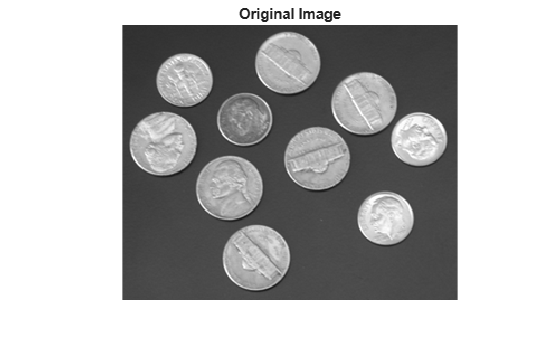Specify an initial contour surrounding the objects of interest. Display the contour.

```mask = zeros(size(I)); mask(25:end-25,25:end-25) = 1; imshow(mask) title('Initial Contour Location')```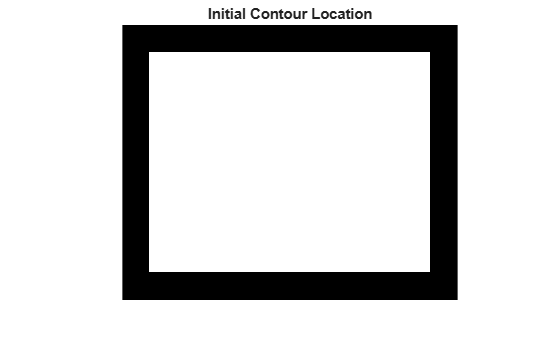Segment the image by using the `activecontour` function. By default, the function evolves the segmentation through 100 iterations.

`bw = activecontour(I,mask);`

Display the result. After 100 iterations, objects are not fully segmented from the background because the original contour is not close to the object boundaries.

```imshow(bw) title('Segmented Image, 100 Iterations')```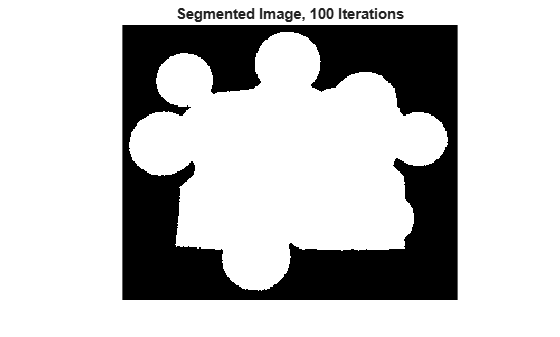To continue evolving the segmentation, increase the number of iterations. After 300 iterations, objects are fully segmented from the background.

```bw = activecontour(I,mask,300); imshow(bw) title('Segmented Image, 300 Iterations')```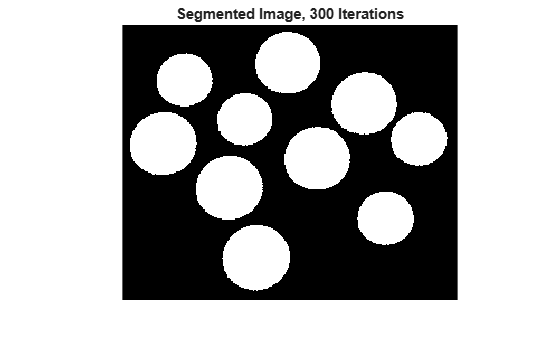Read and display a grayscale image.

```I = imread('toyobjects.png'); imshow(I)```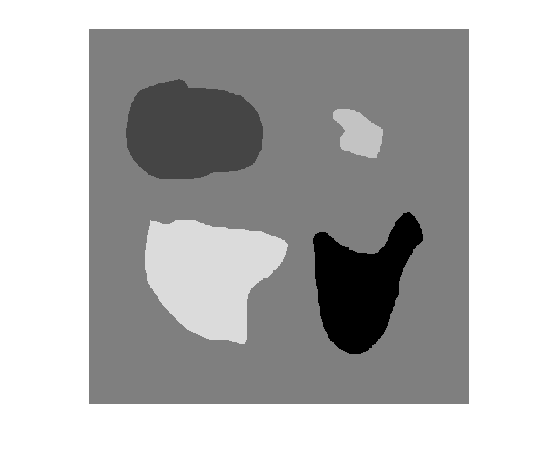Draw an initial contour close to the object of interest by using the `drawrectangle` function. After drawing the contour, create a mask by using the `createMask` function.

`r = drawrectangle;`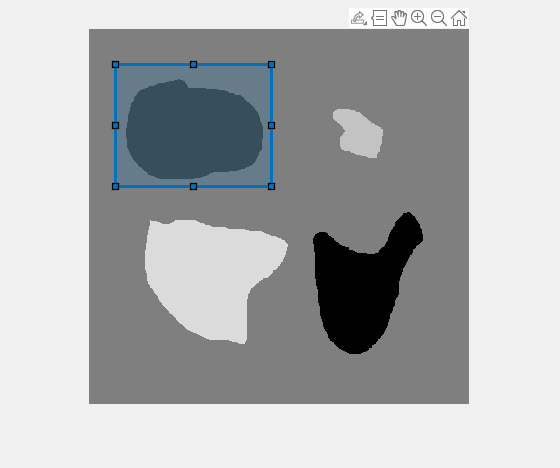`mask = createMask(r);`

Segment the image using the `'edge'` method and 200 iterations.

`bw = activecontour(I,mask,200,'edge');`

Display the final contour over the original image in red.

```hold on; visboundaries(bw,'Color','r'); ```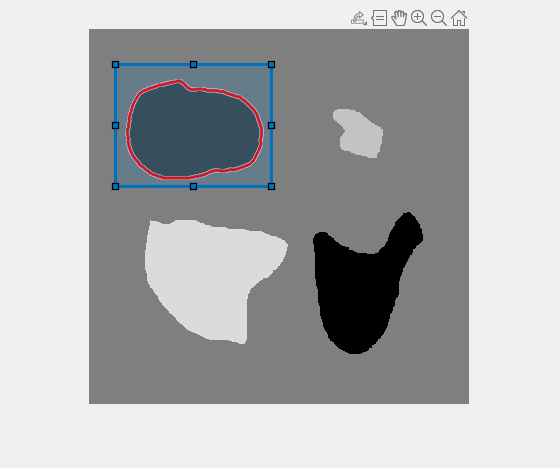Display the result of the segmentation over the original image. The object in the foreground has a blue color.

```figure imshow(labeloverlay(I,bw));```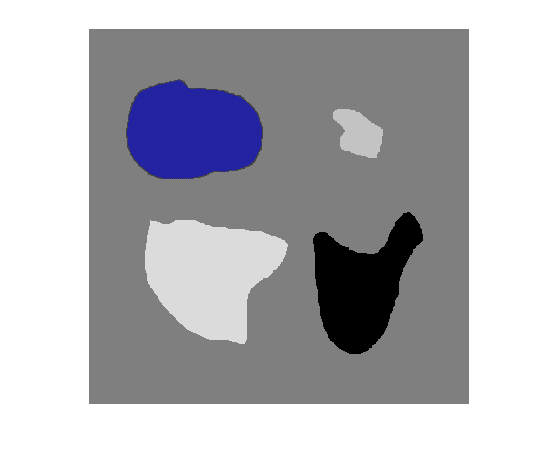Load 3-D volumetric image data, removing the singleton dimension.

```D = load('mri.mat'); A = squeeze(D.D);```

Create 2-D mask for initial seed points.

```seedLevel = 10; seed = A(:,:,seedLevel) > 75; figure imshow(seed)```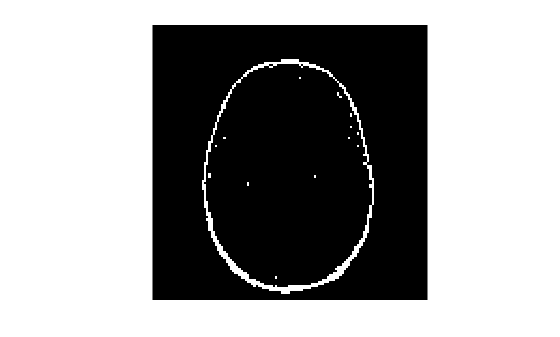Create an empty 3-D seed mask and put the seed points into it.

```mask = zeros(size(A)); mask(:,:,seedLevel) = seed;```

Perform the segmentation using active contours, specifying the seed mask.

`bw = activecontour(A,mask,300);`

Display the 3-D segmented image.

```figure; p = patch(isosurface(double(bw))); p.FaceColor = 'red'; p.EdgeColor = 'none'; daspect([1 1 27/128]); camlight; lighting phong```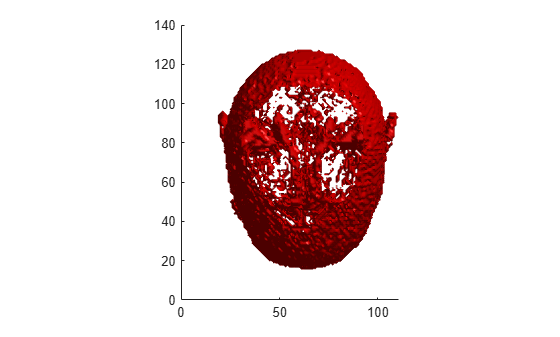## Input Arguments

collapse all

Image to segmented, specified as a 2-D numeric matrix or 3-D numeric array.

Data Types: `single` | `double` | `int8` | `int16` | `int32` | `uint8` | `uint16` | `uint32`

Initial contour at which the evolution of the segmentation begins, specified as a binary image of the same size as `A`. For 2-D and 3-D grayscale images, the size of `mask` must match the size of the image `A`. For color and multi-channel images, `mask` must be a 2-D logical array where the first two dimensions match the first two dimensions of the image `A`.

You can create a mask interactively by using ROI objects. For example, draw a polygonal ROI by using the `drawpolygon` function, then create a mask from the ROI by using the `createMask` function.

Data Types: `logical`

Maximum number of iterations to perform in evolution of the segmentation, specified as a positive integer. `activecontour` stops the evolution of the active contour when it reaches the maximum number of iterations. `activecontour` also stops the evolution if the contour position in the current iteration is the same as the contour position in one of the most recent five iterations.

If the initial contour position (specified by `mask`) is far from the object boundaries, specify larger values of `n` to achieve desired segmentation results.

Data Types: `double`

Active contour method used for segmentation, specified as `'Chan-Vese'` or `'edge'`. The Chan-Vese region-based energy model is described in . The edge-based model, similar to geodesic active contours, is described in .

For RGB images, the method must be `'Chan-Vese'`.

Data Types: `char` | `string`

### Name-Value Arguments

Specify optional pairs of arguments as `Name1=Value1,...,NameN=ValueN`, where `Name` is the argument name and `Value` is the corresponding value. Name-value arguments must appear after other arguments, but the order of the pairs does not matter.

Before R2021a, use commas to separate each name and value, and enclose `Name` in quotes.

Example: `'SmoothFactor',1.5`

Degree of smoothness or regularity of the boundaries of the segmented regions, specified as the comma-separated pair consisting of `'SmoothFactor'` and a positive number. Higher values produce smoother region boundaries but can also smooth out finer details. Lower values produce more irregularities (less smoothing) in the region boundaries but allow finer details to be captured. The default smoothness value is `0` for the `'Chan-Vese'` method and `1` for the `'edge'` method.

Example: `'SmoothFactor',1.5`

Data Types: `single` | `double` | `int8` | `int16` | `int32` | `uint8` | `uint16` | `uint32`

Tendency of the contour to grow outwards or shrink inwards, specified as the comma-separated pair consisting of `'ContractionBias'` and a numeric scalar. Positive values bias the contour to shrink inwards (contract). Negative values bias the contour to grow outwards (expand). This parameter does not guarantee that the contour contracts or expands. It is possible that even with a positive value for this parameter, the contour could actually expand. However, by specifying a bias, you slow the expansion when compared to an unbiased contour. Typical values for this parameter are between -1 and 1. The default contraction bias is `0` for the `'Chan-Vese'` method and `0.3` for the `'edge'` method.

Example: `'ContractionBias',0.4`

Data Types: `single` | `double` | `int8` | `int16` | `int32` | `uint8` | `uint16` | `uint32`

## Output Arguments

collapse all

Segmented image, returned as a binary image of the same size as the input image `A`. The foreground is white (logical true) and the background is black (logical false).

## Tips

• `activecontour` uses the boundaries of the regions in `mask` as the initial state of the contour from where the evolution starts. Holes in the mask can cause unpredictable results. Use `imfill` to fill any holes in the regions in `mask`.

• If a region touches the image borders, then `activecontour` removes a single-pixel layer from the region, before further processing, so that the region does not touch the image border.

• To get faster and more accurate results, specify an initial contour position that is close to the desired object boundaries, especially for the `'edge'` method.

• For the `'edge'` method, the active contour is naturally biased towards shrinking inwards (collapsing). In the absence of any image gradient, the active contour shrinks on its own. Conversely, with the `'Chan-Vese'` method, where the contour is unbiased, the contour is free to either shrink or expand based on the image features.

• To achieve an accurate segmentation with the `'edge'` method, specify an initial contour that lies outside the boundaries of the object. The active contour with the `'edge'` method is biased to shrink, by default.

• If object regions are of significantly different grayscale intensities, then the `'Chan-Vese'` method  might not segment all objects in the image. For example, if the image contains objects that are brighter than the background and some that are darker, the `'Chan-Vese'` method typically segments out either the dark or the bright objects only.

## Algorithms

`activecontour` uses the Sparse-Field level-set method, similar to the method described in , for implementing active contour evolution.

 T. F. Chan, L. A. Vese, Active contours without edges. IEEE Transactions on Image Processing, Volume 10, Issue 2, pp. 266-277, 2001.

 V. Caselles, R. Kimmel, G. Sapiro, Geodesic active contours. International Journal of Computer Vision, Volume 22, Issue 1, pp. 61-79, 1997.

 R. T. Whitaker, A level-set approach to 3d reconstruction from range data. International Journal of Computer Vision, Volume 29, Issue 3, pp. 203-231, 1998.